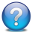## Pages

### Probability Distributions (I)

Probability distributions

• A probability distribution describes how the values of a random variable are distributed.
• It assigns a probability to each possible outcome of a process or experiment that is assumed random. The random variable can be continuous or discrete.
• Probability distributions can be very useful because, since the characteristics of each distribution are well understood, they can be used to, using a sample of observations, make statistical inferences on the entire population.
• A probability distribution can be specified in a number of ways:
• Through a probability density function (probability mass function)
• Through a cumulative distribution function (survival function)
• Through a hazard function
• Through a characteristic function
• Some common distributions include:
• Binomial distribution: dbinom()
• The collection of possible outcomes of a coin toss [H|T] follow a
• Cauchy distribution: dcauchy()
• Chi-squared distribution: dchisq()
• Exponential distribution: dexp()
• F distribution: df()
• Gamma distribution: dgamma()
• Hypergeometric distribution: dhyper()
• Log-normal distribution: dlnorm()
• Geometric distribution: dgeom()
• Multinomial distribution: dmultinom()
• Negative binomial distribution: dnbinom()
• Normal distribution: dnorm()
• Poisson distribution: dpois()
• Student's t distribution: dhyper()
• Uniform distribution: dunif()
• Weibull distribution: dweibull()

### If you get: "xterm-256color: Unknown terminal type"

When connecting to a Solaris host, using ssh, from MacOSX or a Linux machine, you may get the message when you try to use vi:

For example, you may try:
$vi instructions.txt xterm-256color: Unknown terminal type I don't know what kind of terminal you are on - all I have is 'xterm-256color'. [Using open mode] "instructions.txt" 25 lines, 2465 characters Instructions to run the Gateway Management utilitySolution? You need to define the TERM environment variable. $ export TERM=xterm
\$ vi instructions.txt